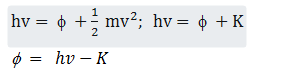# When photons of energy hv fall on an aluminium plate (of work function E0), photoelectrons of maximum kinetic energy K are ejected. If the frequency of the radiation is doubled, the maximum kinetic energy of the ejected photoelectrons will be         (a)    2K         (b)    K         (c)    K + hv         (d)    K + E09 years ago

(c)

Applying Einstein''s formula for photoelectricityIf we use 2v frequency then let the kinetic energy becomes K''

So,

h. 2v = f + K''

2hv = hv – K + K''

K''= hv + K